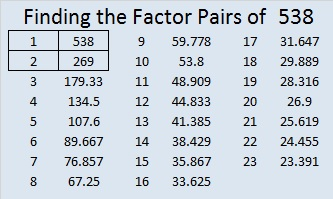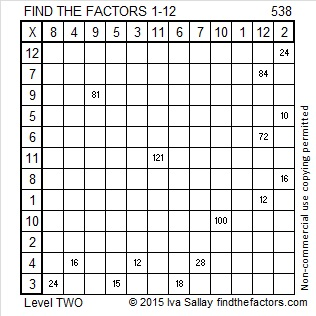# 538 and Level 2

538 is the hypotenuse of the Pythagorean triple 138-520-538. Can you find the greatest common factor of those three numbers?Print the puzzles or type the solution on this excel file: 12 Factors 2015-06-29

—————————————————————————————————

• 538 is a composite number.
• Prime factorization: 538 = 2 x 269
• The exponents in the prime factorization are 1 and 1. Adding one to each and multiplying we get (1 + 1)(1 + 1) = 2 x 2 = 4. Therefore 538 has exactly 4 factors.
• Factors of 538: 1, 2, 269, 538
• Factor pairs: 538 = 1 x 538 or 2 x 269
• 538 has no square factors that allow its square root to be simplified. √538 ≈ 23.194827—————————————————————————————————